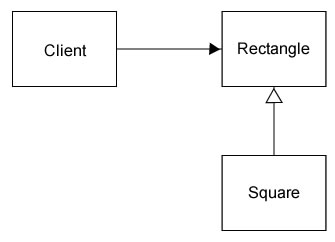## SOLID inheritance

The Liskov Substitution Principle

Created by Jeroen De Dauw for Wikimedia Deutschland

## Original definition

If for each object o1 of type S there is an object o2 of type T such that for all programs P defined in terms of T, the behaviour of P is unchanged when o1 is substituted for o2 then S is a subtype of T.Users expecting a Type can be given a Subtype,
and then use it as a Type without blowing up

All methods need to be there

``````
interface Logger {
public function log( \$stuff );
public function notice( \$stuff );
public function warning( \$stuff );
}
``````
``````
class FooBarLogger implements Logger {
public function log( \$stuff ) {}
public function notice( \$stuff ) {}
// Who needs warnings anyway?
}
``````
``````
function doStuff( Logger \$logger ) {
\$logger->warning( 'Stuff is being done. Slack is decreasing' );
}
``````
``````
doStuff( new FooBarLogger() ); // boom
``````

Contract goes beyond type

``````
interface Logger {
public function log( \$stuff );
public function notice( \$stuff );
public function warning( \$stuff );
}
``````
``````
public function log( \$stuff ) {
if ( strlen( \$stuff ) > 140 ) { throw new NotLspException(); }
}
// ...
}
``````
• Input requirements need to be at least as general
• Output requirements need to be at least as strict

## Obvious!

Or not?

### The classic example

``````
class Rectangle {
private \$width;
private \$height;
private \$topLeftCorner;

public function getWidth();
public function getHeight();

public function setWidth( \$width );
public function setHeight( \$height );

public function getArea();
}
``````

What is the relationship?

Square is a rectangle

=> inheritance!### Implementation

``````
class Rectangle {
private \$width;
private \$height;
private \$topLeftCorner;

public function getWidth();
public function getHeight();

public function setWidth( \$width );
public function setHeight( \$height );

public function getArea();
}

class Square extends Rectangle {}
``````

Too many fields - whatever, enough memory anyway!

But what about `setHeight` and `setWidth`?
Width and height need to be the same

### Fixing the problem

``````
class Square extends Rectangle {
public function setWidth( \$width ) {
\$this->width = \$width;
\$this->height = \$width;
}

public function setHeight( \$height ) {
\$this->width = \$height;
\$this->height = \$height;
}
}
``````

Still somewhat odd

Though behaves like a square

``````
function f( Rectangle \$rectangle ) {
\$rectangle->setWidth( 10 );
\$rectangle->setHeight( 5 );

assert( \$rectangle->getArea() === 50 );
}
``````
``````
f( new Square() ); // Boom
``````

Have fun debugging!

Always code as if the person who ends up maintaining your code is a violent psychopath who knows where you live. --John Woods

How to fix this mess?

### Fixing the fix new problem

``````
function f( Rectangle \$r ) {
if ( \$r instanceof Square ) {
// ...
}
else { // Assume we got a Rectangle
// ...
}
}
``````

OCP violation!

`f` now dependent on `Square`

Shotgun Surgery

What are boundaries?

### Third try is a charm!

Let `Rectangle` extend `Square`

``````
class Square {
private \$side;
}
``````
``````
class Rectangle extends Square {
private \$otherSide;
}
``````
``````
\$square->setSide( 5 );
``````
``````
assert( \$square->getArea() === 25 ); // boom if \$square instanceof Rectangle
``````

### The actual solution

Square and Rectangle are different, don't subtype!

Never pass a Square into a method expecting Rectangle

Square is not a Rectangle

### WTF?

Squares are not rectangles?!

Yeah, they are rectangles

Squares are rectangles

``````
// This is not a square though
class Square {
// ...
}
``````

It is code that represents a square

### Representatives

Do not share the relationships of the things they represent

Integer is a real number

Real number is a complex number

Complex number contains two real number

Makes perfect sense.
Free beer for who implements this and successfully instantiates a complex number

``````
class ComplexNumber {
private \$realPart;
private \$imaginaryPart;

function __construct( RealNumber \$real, RealNumber \$imaginary ) {
\$this->realPart = \$real;
\$this->imaginaryPart = \$imaginary;
}
public function getRealPart(): RealNumber {
return \$this->realPart;
}
public function getImaginaryPart(): RealNumber {
return \$this->imaginaryPart;
}
}
``````
``````
class RealNumber extends ComplexNumber {

public function __construct( float \$value ) {
parent::__construct(
new RealNumber( \$value ),
new RealNumber( 0 )
);
}

}
``````

Class T

Class S extends T

List<S> is not a subtype of List<T>

Cannot pass list<Square> to function expecting list<Shape>

``````
\$squares = new List<Square>();

function f( List<Shape> \$list ) { /* ... */ }
``````
``````
f( \$squares );
``````
``````
function f( List<Shape> \$list ) {
}
``````

The relationship is again not carried over

Questions?

These slides: bit.ly/solid-lsp

Liskov substitution principle (WP)

LSP (Robert C Martin, pdf)